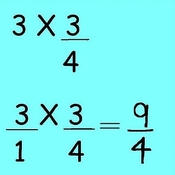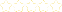+# Multiplying Fractions By Whole Numbers

Owner: Rachel Casto
##### Description:
Operations and Algebraic Thinking ⎯ 4.OA  A. Use the four operations with whole numbers to solve problems. 1. Interpret a multiplication equation as a comparison, e.g., interpret 35 = 5 × 7 as a statement that 35 is 5 times as many as 7 and 7 times as many as 5. Represent verbal statements of multiplicative comparisons as multiplication equations. Number and Operations—Fractions 4.NF  B. Build fractions from unit fractions by applying and extending previous understandings of operations on whole numbers.  4. Apply and extend previous understandings of multiplication to multiply a fraction by a whole number. a. Understand a fraction a/b as a multiple of 1/b. For example, use a visual fraction model to represent 5/4 as the product 5 × (1/4), recording the conclusion by the equation 5/4 = 5 × (1/4). b. Understand a multiple of a/b as a multiple of 1/b, and use this understanding to multiply a fraction by a whole number. For example, use a visual fraction model to express 3 × (2/5) as 6 × (1/5), recognizing this product as 6/5. (In general, n × (a/b) = (n × a)/b.) c. Solve word problems involving multiplication of a fraction by a whole number, e.g., by using visual fraction models and equations to represent the problem. For example, if each person at a party will eat 3/8 of a pound of roast beef, and there will be 5 people at the party, how many pounds of roast beef will be needed? Between what two whole numbers does your answer lie? NOTE: Grade 4 expectations in this domain are limited to fractions with denominators 2, 3, 4, 5, 6, 8, 10, 12, and 100.
2 Tutorials in this Playlist

#### Multiply Whole by Mixed Number

Author:
Rating:(0)

#### Multiply Whole by Fraction

Author:
Rating:(1)